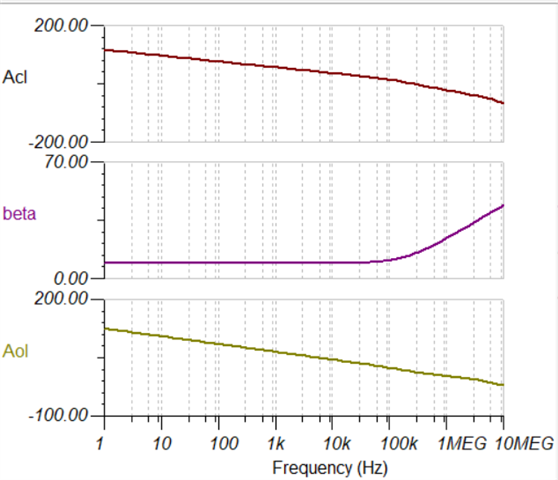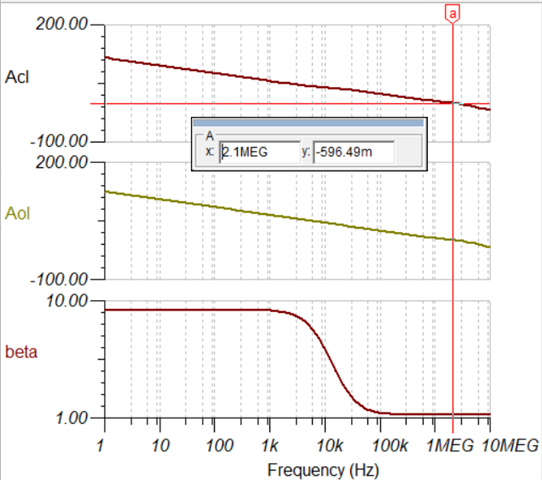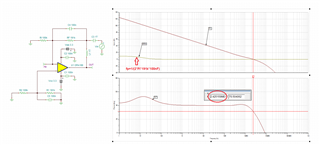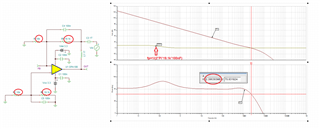If you have a related question, please click the "Ask a related question" button in the top right corner. The newly created question will be automatically linked to this question.

# OPA4188: Effect of input capacitance

Part Number: OPA4188

Hi,

The below LPF is built using OPA4188,  I have following questions

1. The pole in feedback because of input capacitance (Ccm, Cd, Cstray and Req = RF||Rin), causes the zero in 1/beta plot as show at ~130kHz, plots are shown below.

When CF is added to counter the pole in feedback path, zero is added at ~8.3kHz (second plot). It reduces the overall bandwidth of close loop to the placement of zero in the feedback path, is the interpretation correct?2. When I scale down the resistor values to achieve same gain from RF 191k to 19.1k and Rin 100k to 10k, I receive similar results except pole in the feedback path will have different frequency ~1.3MHz. What other advantages I get if scale down the input and feedback resistor?

3. while doing the stability analysis (both the inputs are grounded), then why feedback path or gain is showing as of non inverting configuration?

Regards,

SunneyOPA4188_SIM.TSC

The pole in feedback because of input capacitance (Ccm, Cd, Cstray and Req = RF||Rin), causes the zero in 1/beta plot as show at ~130kHz, plots are shown below.

The RF||CF cause a pole and not a zero - see below.

When CF is added to counter the pole in feedback path, zero is added at ~8.3kHz (second plot). It reduces the overall bandwidth of close loop to the placement of zero in the feedback path, is the interpretation correct?

No, this is not correct. 100nF||191k cause a pole at 8Hz - see below - where the dc gain of G=2.91 (9.3dB) get rolled off to zero (due to CF becoming a short).2. When I scale down the resistor values to achieve same gain from RF 191k to 19.1k and Rin 100k to 10k, I receive similar results except pole in the feedback path will have different frequency ~1.3MHz. What other advantages I get if scale down the input and feedback resistor?The effective bandwidth in both cases is around 2.4MHz (need to use 1TH and 1TF instead of 100k).  The only advantage would be a lower thermal noise of resistors.

3. while doing the stability analysis (both the inputs are grounded), then why feedback path or gain is showing as of non inverting configuration?

Effective bandwidth of configuration is determined by a noise gain (and not signal gain) - a gain taken from the perspective of non-inverting input terminal.